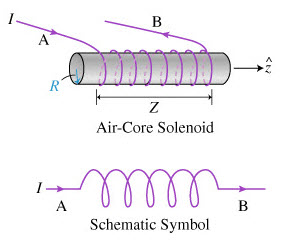Inductors

Inductors

An inductor is also referred to as an AC resistor which stores electrical energy in the form of magnetic energy. It resists the changes in the current and the standard unit of inductance is Henry. The capability of producing magnetic lines is referred to as inductance. The inductance of the inductor is given as L= (µ.K.N2.S)/I. Where, ‘L’ is inductance, ‘µ’ is Magnetic permeability, ‘K’ is a magnetic coefficient, ‘S’ is the cross-section area of the coil, ‘N’ is the number of turns of the coils, And ‘I’ is the length of the coil in the axial direction.Inductor Components